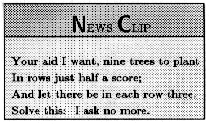Video:
Independent events; probability of an intersection  (time: 8:03)

Tree diagrams (time: 6:07)
See the whole entry

## Homework Hints 13-5

### Note: Homework Hints are given only for the Level 1 and Level 2 problems.

However, as you go through the book be sure you look at all the examples in the text. If you need hints for the Level 3 … See the whole entry

## Homework Hints 13-4

### Note: Homework Hints are given only for the Level 1 and Level 2 problems.

However, as you go through the book be sure you look at all the examples in the text. If you need hints for the Level 3 … See the whole entry

## 13.4 Outline

1. Keno games
2. Independent events
1. independent
2. dependent
3. Probability of an intersection
1. multiplication property of probability
2. birthday problem
4. Probability of a union
2. probability of independent events
5. Drawing with and without replacement
6. Stochastic processes and tree diagrams
See the whole entry

## 12.1 Outline

1. Election problem
1. tree diagram
2. fundamental counting principle
3. arrangement
4. ordered pair/ordered triple
5. define permutation
6. notation for permutations
7. evaluating permutations
2. Factorial
1. definition
2. multiplication property of factorials
3. count-down property
4. permutation formula
3. Distinguishable permutations
1. indistinguishable items
2. distinguishable items
3. formula for distinguishable permutations
See the whole entry

## Individual Research Projects Section 9.3

### Project 9.5

Prepare a classroom demonstration of topology by drawing geometric figures on a piece of rubber inner tube. Demonstrate to the class various ways in which these figures can be distorted.

### Project 9.6The problem shown in the News … See the whole entry

Videos:
Kruskal’s Algorithm (time: 2:40)

This is a rather foolish introduction to spanning trees  (time: 1:39)
See the whole entry

## Homework Hints 9-2

### Note: Homework Hints are given only for the Level 1 and Level 2 problems.

However, as you go through the book be sure you look at all the examples in the text. If you need hints for the Level 3 … See the whole entry

## 9.2 Outline

1. Trees
1. definition
2. spanning tree
2. Minimum spanning trees
1. weight
2. weighted graph
3. definition
4. Kruskal’s algorithm
5. number-of-vertices-and-edges-in-a-tree theorem

## 9.2 Essential Ideas

A tree is a graph which is connected and has no circuits. A tree that is created from another … See the whole entry